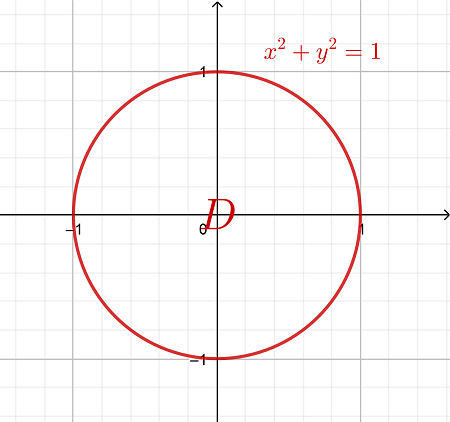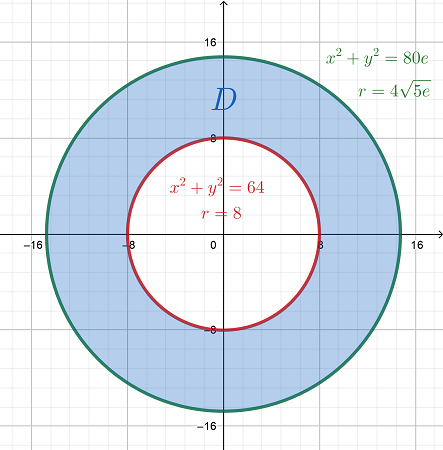# Determine the diameter of a hole that is drilled vertically through the center of the solid...

## Question:

Determine the diameter of a hole that is drilled vertically through the center of the solid bounded by the graphs of the equations {eq}z=20e-\frac{(x2+y2)}{4} {/eq} , {eq}z = 0 {/eq}, and {eq}x2 + y2 = 64 {/eq} if one-tenth of the volume of the solid is removed. (Round your answer to four decimal places.)

## Volume of a Solid:

Consider the solid {eq}S {/eq} between the surfaces {eq}F(x,y) \ge G(x,y) {/eq}. Additionally, let {eq}D {/eq} be the region on the xy-plane that is the projection of the solid on the same plane.

The volume of the said integral, which is usually given by a triple integral, can then be expressed as the double integral:

$$Volume = \iiint \limits_S dV = \iint \limits_D \left[ F(x,y) - G(x,y) \right] \,dA$$

Let {eq}E {/eq} be the given solid that is bounded by the graphs of {eq}z = 20e - \frac{x^2 + y^2}{4} \,,\, z = 0 \text{ and } x^2 + y^2 = 64 {/eq}. Also, let {eq}D {/eq} the be region on the xy-plane under the said solid.Determine the intersection of the paraboloid with the xy-plane {eq}(z = 0) {/eq}:

{eq}\begin{align*} &z = 20e - \frac{x^2 + y^2}{4} = 0 \\ &\Rightarrow \frac{x^2 + y^2}{4} = 20e \\ &\Rightarrow x^2 + y^2 = 80e \end{align*} {/eq}

Since both the cylinder {eq}x^2 + y^2 = 64 {/eq} and the paraboloid have circular intersection with the xy-plane, express the equations of these intersections in their polar forms:

{eq}\begin{align*} &x^2 + y^2 = 80e & \text{[Use the identity } x^2 + y^2 = r^2 ] \\ &\Rightarrow r^2 = 80e & \text{[Take the positive root of } r ] \\ &\Rightarrow r = 4 \sqrt{5e} \\ \\ &x^2 + y^2 = 64 \\ &\Rightarrow r^2 = 64 \\ &\Rightarrow r = 8 \end{align*} {/eq}

Therefore, the region {eq}D {/eq} under the given solid {eq}E {/eq} is defined in polar coordinates as {eq}\left\{\, (r,\theta) \,|\, 8 \le r \le 4\sqrt{5e} \text{ and } 0 \le \theta \le 2\pi \,\right\} {/eq}.Therefore, the volume of the solid {eq}E {/eq} bounded above by {eq}z = 80e - \frac{x^2 + y^2}{4} {/eq} and below by the region {eq}D {/eq} on the xy-plane is given by the double integrals:

{eq}\begin{align*} Volume_E &= \iint \limits_D \left[ \left( 80e - \frac{x^2 + y^2}{4} \right) - 0 \right] \,dA & \text{[Covert the integrand into its polar form and evaluate as iterated integrals in polar coordinates]} \\ &= \int_0^{2\pi} \int_8^{4\sqrt{5e}} \left( 80e - \frac{r^2}{4} \right) \cdot r \,dr \,d\theta & \text{[Where } dA = r \,dr\,d\theta ] \\ &= \int_0^{2\pi} \int_8^{4\sqrt{5e}} \left( 80er - \frac{r^3}{4} \right) \,dr \,d\theta & \text{[Integrate with respect to } r \text{]} \\ &= \int_0^{2\pi} \left. \left( 40er^2 - \frac{r^4}{16} \right) \right|_8^{4\sqrt{5e}} \,d\theta & \text{[Fundamental Theorem of Calculus]} \\ &= \int_0^{2\pi} \left( \left[ 40e \left( 4\sqrt{5e} \right)^2 - \frac{\left( 4\sqrt{5e} \right)^4}{16} \right] - \left[ 40e(8)^2 - \frac{8^4}{16} \right] \right) \,d\theta \\ &= \int_0^{2\pi} \left[ \left( 3200e^2 - 400e^2 \right) - ( 2560e - 256) \right] \,d\theta \\ &= \int_0^{2\pi} ( 2800e^2 - 2560e + 256 ) \,d\theta & \text{[Integrate with respect to } \theta \text{]} \\ &= \left. 16 ( 175e^2 - 160e + 16 ) \theta \right|_0^{2\pi} & \text{[Fundamental Theorem of Calculus]} \\ &= 16 ( 175e^2 - 160e + 16 ) (2\pi - 0 ) \\ Volume_E &= \boxed{ 32\pi ( 175e^2 - 160e + 16 ) } & \text{[Volume of the Solid } E ] \end{align*} {/eq}

Let {eq}S {/eq} be the solid that is to be drilled through the center of the solid {eq}E {/eq} such that its volume is one-tenth of the volume of the solid {eq}E {/eq}. Also, let {eq}R {/eq} be the region on the xy-plane under this solid {eq}S {/eq}.

Furthermore, let {eq}a \in \left[ 8 , 4\sqrt{5e} \right] {/eq} be the outer-diameter of the solid {eq}S {/eq} and planar region {eq}R {/eq}. Then, the solid {eq}S {/eq} is bounded above by the paraboloid {eq}z = 80e - \frac{x^2 + y^2}{4} = 80e - \frac{r^2}{4} {/eq} and below by the region {eq}R = \left\{\, (r,\theta) \,|\, 8 \le r \le a \text{ and } 0 \le \theta \le 2\pi \,\right\} {/eq}.

Solve for the volume of this solid {eq}S {/eq} using double integrals:

{eq}\begin{align*} Volume_S &= \int_0^{2\pi} \int_8^a \left( 80e - \frac{r^2}{4} \right) \cdot r \,dr \,d\theta \\ &= \int_0^{2\pi} \int_8^a \left( 80er - \frac{r^3}{4} \right) \,dr \,d\theta & \text{[Integrate with respect to } r \text{]} \\ &= \int_0^{2\pi} \left. \left( 40er^2 - \frac{r^4}{16} \right) \right|_8^a \,d\theta & \text{[Fundamental Theorem of Calculus]} \\ &= \int_0^{2\pi} \left( \left[ 40e(a)^2 - \frac{a^4}{16} \right] - \left[ 40e(8)^2 - \frac{8^4}{16} \right] \right) \,d\theta \\ &= \int_0^{2\pi} \left[ \left( 40a^2e - \frac{a^4}{16} \right) - ( 2560e - 256) \right] \,d\theta \\ &= \int_0^{2\pi} \left( - \frac{a^4}{16} + 40a^2e - 2560e + 256 \right) \,d\theta & \text{[Integrate with respect to } \theta \text{]} \\ &= \left. \left( - \frac{a^4}{16} + 40a^2e - 2560e + 256 \right) \right|_0^{2\pi} & \text{[Fundamental Theorem of Calculus]} \\ &= \left( - \frac{a^4}{16} + 40a^2e - 2560e + 256 \right) (2\pi - 0) \\ Volume_S &= \boxed{ 2\pi \left( - \frac{a^4}{16} + 40a^2e - 2560e + 256 \right) } & \text{[Volume of the Drilled-Out Solid } S ] \end{align*} {/eq}

Solve for the outer diameter {eq}a {/eq} of the solid {eq}S {/eq} using the volumes of both solids:

{eq}\begin{align*} &Volume_S = \frac{1}{10} Volume_E & \text{[Substitute the calculated volumes of the solids]} \\ &\Rightarrow 2\pi \left( - \frac{a^4}{16} + 40a^2e - 2560e + 256 \right) = \frac{32\pi }{10} ( 175e^2 - 160e + 16 ) & \text{[Solve for } a ] \\ &\Rightarrow - \frac{a^4}{16} + 40ea^2 - 2560e + 256 = \frac{1}{10} (2800e^2 - 2560e + 256) \\ &\Rightarrow - a^4 + 640ea^2 - 40960e + 4096 = 4480e^2 - 4096e + \frac{2048}{5} \\ &\Rightarrow a^4 - 640ea^2 + \left( 4480e^2 + 36864e - \frac{18432}{5} \right) = 0 & \left[ \text{Solve using Quadratic Formula. Note that } 8 \le a \le 4\sqrt{5e} \right] \\ &\Rightarrow a = \sqrt{ \frac{ 3200e - \sqrt{9792000e^2 - 3686400e + 368640} }{10} } \\ &\Rightarrow \boxed{ a \approx 8.8321 } & \boxed{ \text{Outside Diameter of the Drilled Hole} } \end{align*} {/eq}